# High School Math : Graphing

## Example Questions

### Example Question #1 : Graphing

The vertices of a triangle are given by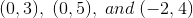.  The triangle is rotated about the origin bydegrees clockwise.  What are the new coordinates?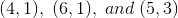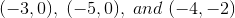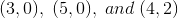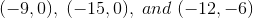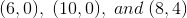Explanation:

The coordinates form a triangle in the second quadrant with a side along the y-axis.  The rotation about the origin bydegrees clockwise results in a triangle in the first quadrant with a side along the x-axis.  There are two responses that give triangles along the x-axis:andA rotation and a dialation by a factor ofis given by, so the correct answer is